# RD Sharma Solutions for Class 11 Chapter 1 - Sets Exercise 1.3

This exercise mainly deals with problems based on types of sets, which include empty set, singleton set, finite set, infinite set, equivalent sets and equal sets. From the exam point of view, solutions are prepared using shortcut techniques, which speed up the ability to solve problems. Students may keep the textbook aside and use RD Sharma Class 11 Solutions for revision purpose. Students can refer and download the Pdf from the links provided below.

## Download the Pdf of RD Sharma Solutions for Class 11 Maths Exercise 1.3 Chapter 1 – Sets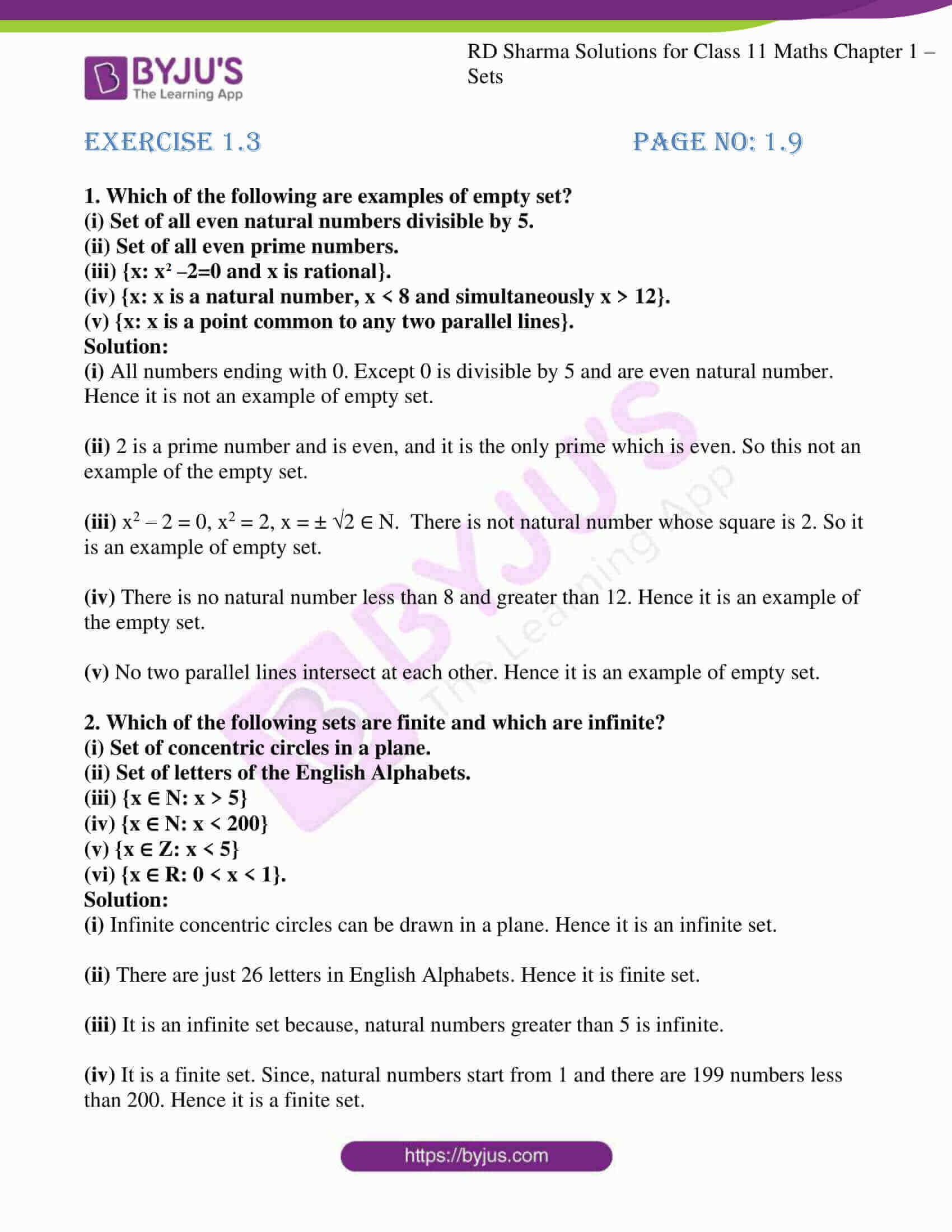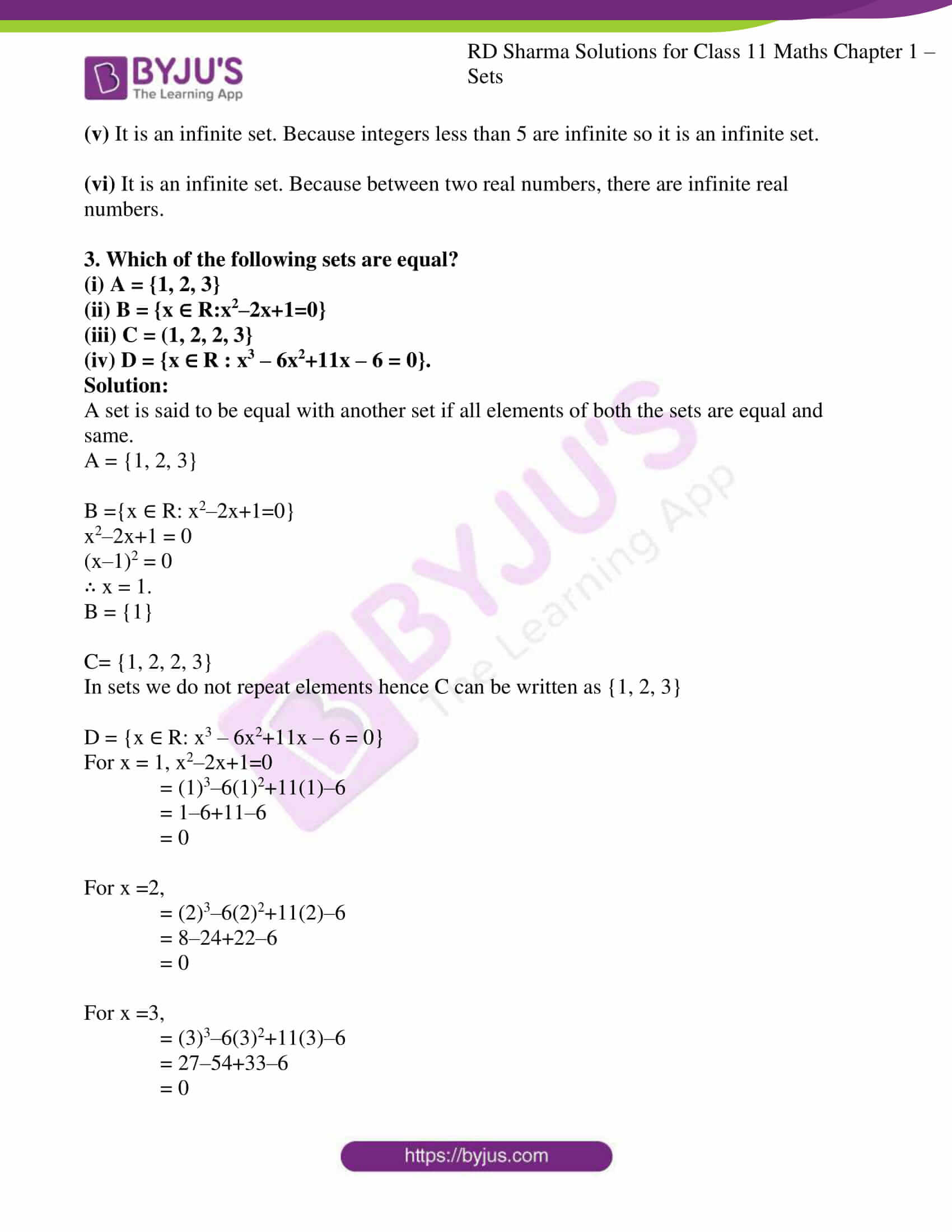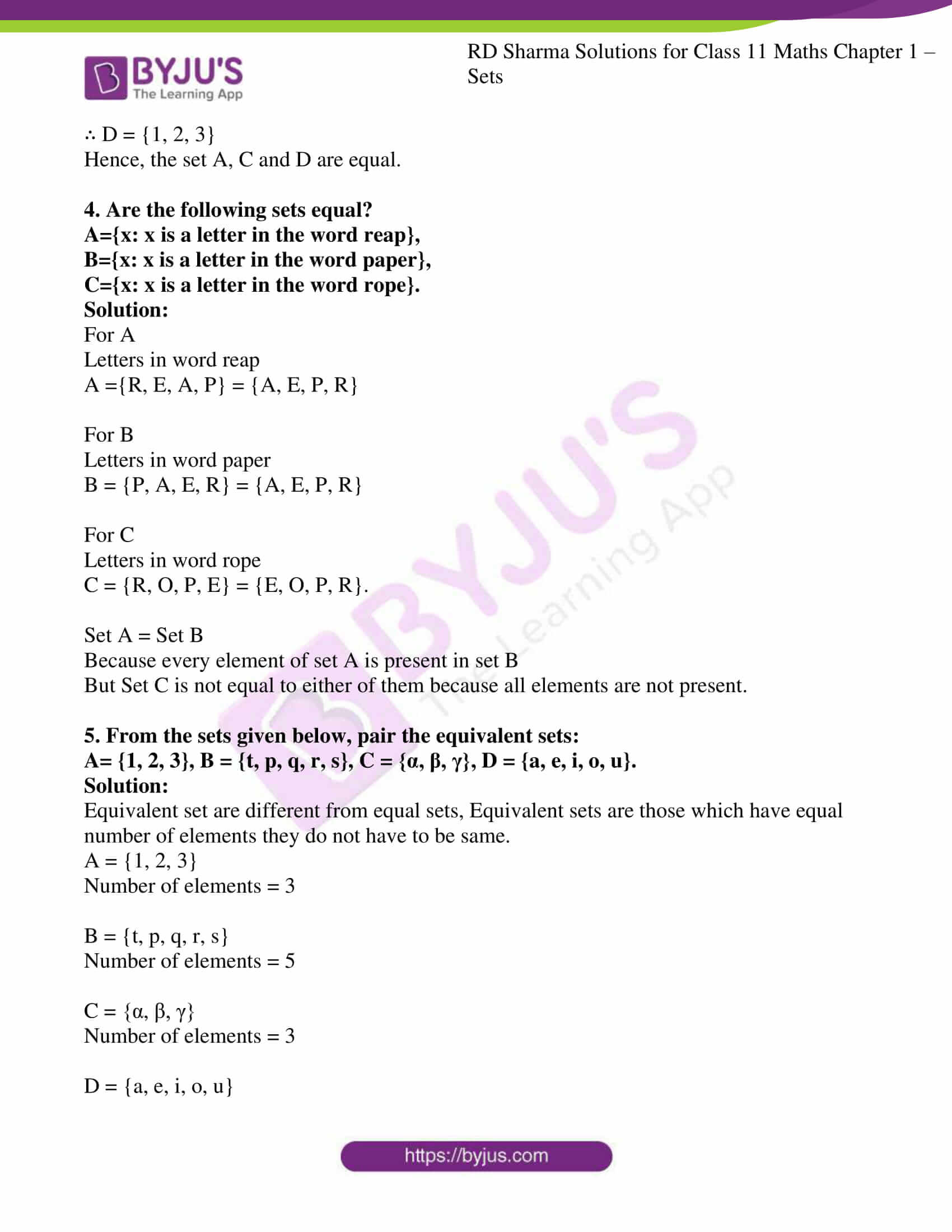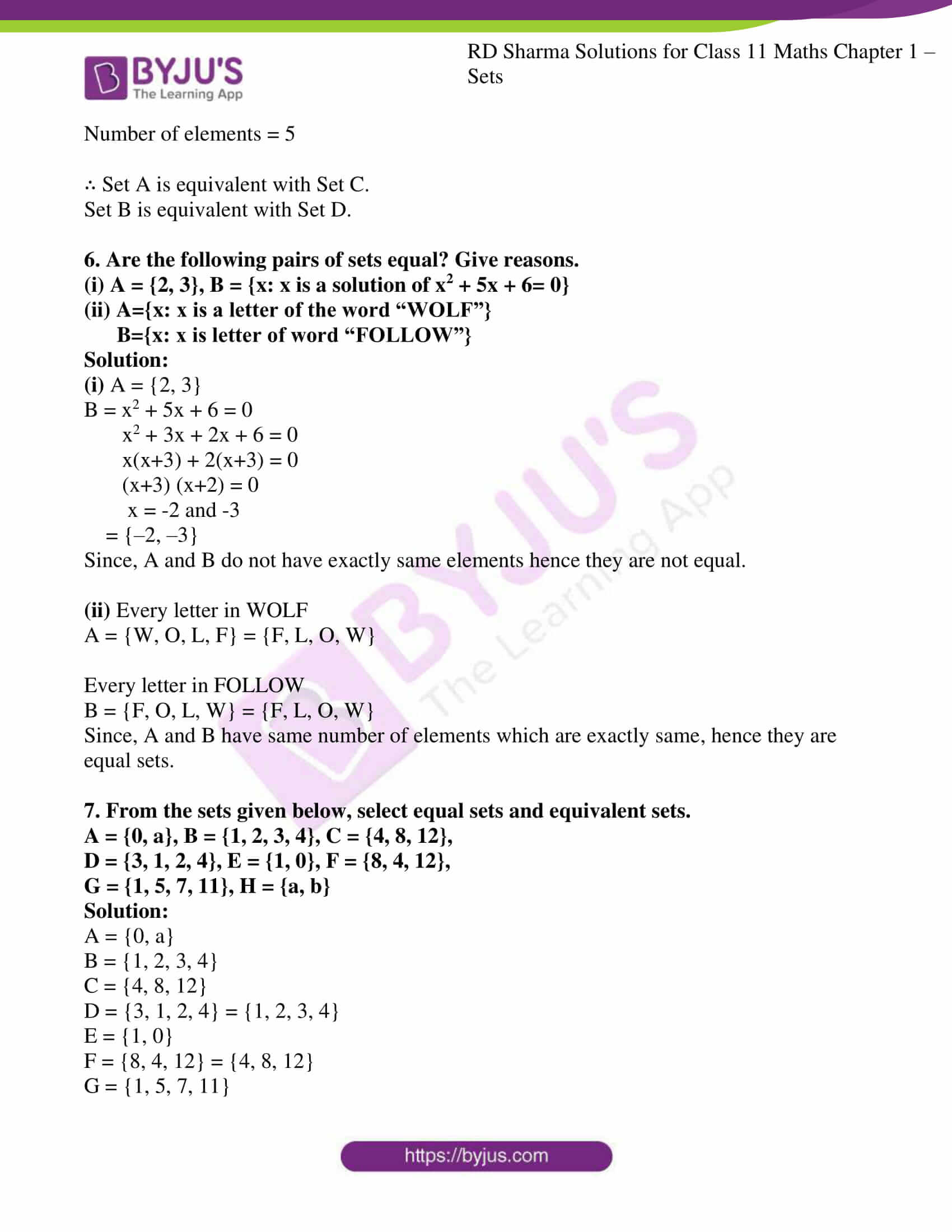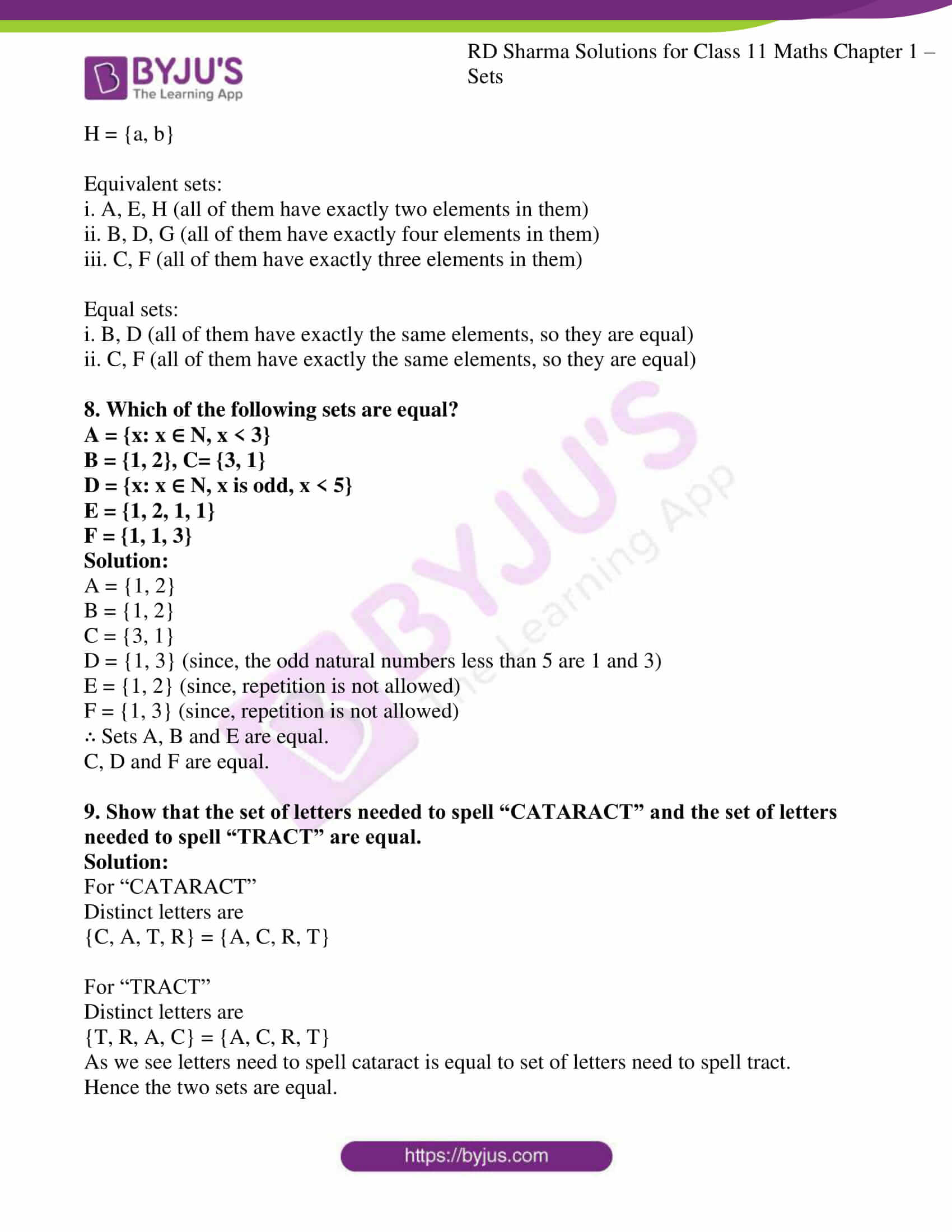### Access answers to RD Sharma Solutions for Class 11 Maths Exercise 1.3 Chapter 1 – Sets

1. Which of the following are examples of empty set?
(i) Set of all even natural numbers divisible by 5.
(ii) Set of all even prime numbers.
(iii) {x: x2–2=0 and x is rational}.
(iv) {x: x is a natural number, x < 8 and simultaneously x > 12}.
(v) {x: x is a point common to any two parallel lines}.

Solution:

(i) All numbers ending with 0. Except 0 is divisible by 5 and are even natural number. Hence it is not an example of empty set.

(ii) 2 is a prime number and is even, and it is the only prime which is even. So this not an example of the empty set.

(iii) x2 – 2 = 0, x2 = 2, x = ± √2 ∈ N. There is not natural number whose square is 2. So it is an example of empty set.

(iv) There is no natural number less than 8 and greater than 12. Hence it is an example of the empty set.

(v) No two parallel lines intersect at each other. Hence it is an example of empty set.

2. Which of the following sets are finite and which are infinite?
(i) Set of concentric circles in a plane.
(ii) Set of letters of the English Alphabets.
(iii) {x ∈ N: x > 5}
(iv) {x ∈ N: x < 200}
(v) {x ∈ Z: x < 5}
(vi) {x ∈ R: 0 < x < 1}.

Solution:

(i) Infinite concentric circles can be drawn in a plane. Hence it is an infinite set.

(ii) There are just 26 letters in English Alphabets. Hence it is finite set.

(iii) It is an infinite set because, natural numbers greater than 5 is infinite.

(iv) It is a finite set. Since, natural numbers start from 1 and there are 199 numbers less than 200. Hence it is a finite set.

(v) It is an infinite set. Because integers less than 5 are infinite so it is an infinite set.

(vi) It is an infinite set. Because between two real numbers, there are infinite real numbers.

3. Which of the following sets are equal?
(i) A = {1, 2, 3}
(ii) B = {x ∈ R:x2–2x+1=0}
(iii) C = (1, 2, 2, 3}
(iv) D = {x ∈ R : x3 – 6x2+11x – 6 = 0}.

Solution:

A set is said to be equal with another set if all elements of both the sets are equal and same.

A = {1, 2, 3}

B ={x ∈ R: x2–2x+1=0}

x2–2x+1 = 0

(x–1)2 = 0

∴ x = 1.

B = {1}

C= {1, 2, 2, 3}

In sets we do not repeat elements hence C can be written as {1, 2, 3}

D = {x ∈ R: x3 – 6x2+11x – 6 = 0}

For x = 1, x2–2x+1=0

= (1)3–6(1)2+11(1)–6

= 1–6+11–6

= 0

For x =2,

= (2)3–6(2)2+11(2)–6

= 8–24+22–6

= 0

For x =3,

= (3)3–6(3)2+11(3)–6

= 27–54+33–6

= 0

∴ D = {1, 2, 3}

Hence, the set A, C and D are equal.

4. Are the following sets equal?
A={x: x is a letter in the word reap},

B={x: x is a letter in the word paper},
C={x: x is a letter in the word rope}.

Solution:

For A

Letters in word reap

A ={R, E, A, P} = {A, E, P, R}

For B

Letters in word paper

B = {P, A, E, R} = {A, E, P, R}

For C

Letters in word rope

C = {R, O, P, E} = {E, O, P, R}.

Set A = Set B

Because every element of set A is present in set B

But Set C is not equal to either of them because all elements are not present.

5. From the sets given below, pair the equivalent sets:
A= {1, 2, 3}, B = {t, p, q, r, s}, C = {α, β, γ}, D = {a, e, i, o, u}.

Solution:

Equivalent set are different from equal sets, Equivalent sets are those which have equal number of elements they do not have to be same.

A = {1, 2, 3}

Number of elements = 3

B = {t, p, q, r, s}

Number of elements = 5

C = {α, β, γ}

Number of elements = 3

D = {a, e, i, o, u}

Number of elements = 5

∴ Set A is equivalent with Set C.

Set B is equivalent with Set D.

6. Are the following pairs of sets equal? Give reasons.
(i) A = {2, 3}, B = {x: x is a solution of x2 + 5x + 6= 0}

(ii) A={x: x is a letter of the word “WOLF”}
B={x: x is letter of word “FOLLOW”}

Solution:

(i) A = {2, 3}

B = x2 + 5x + 6 = 0

x2 + 3x + 2x + 6 = 0

x(x+3) + 2(x+3) = 0

(x+3) (x+2) = 0

x = -2 and -3

= {–2, –3}

Since, A and B do not have exactly same elements hence they are not equal.

(ii) Every letter in WOLF

A = {W, O, L, F} = {F, L, O, W}

Every letter in FOLLOW

B = {F, O, L, W} = {F, L, O, W}

Since, A and B have same number of elements which are exactly same, hence they are equal sets.

7. From the sets given below, select equal sets and equivalent sets.
A = {0, a}, B = {1, 2, 3, 4}, C = {4, 8, 12},
D = {3, 1, 2, 4}, E = {1, 0}, F = {8, 4, 12},
G = {1, 5, 7, 11}, H = {a, b}

Solution:

A = {0, a}

B = {1, 2, 3, 4}

C = {4, 8, 12}

D = {3, 1, 2, 4} = {1, 2, 3, 4}

E = {1, 0}

F = {8, 4, 12} = {4, 8, 12}

G = {1, 5, 7, 11}

H = {a, b}

Equivalent sets:

i. A, E, H (all of them have exactly two elements in them)

ii. B, D, G (all of them have exactly four elements in them)

iii. C, F (all of them have exactly three elements in them)

Equal sets:

i. B, D (all of them have exactly the same elements, so they are equal)

ii. C, F (all of them have exactly the same elements, so they are equal)

8. Which of the following sets are equal?
A = {x: x ∈ N, x < 3}
B = {1, 2}, C= {3, 1}
D = {x: x ∈ N, x is odd, x < 5}
E = {1, 2, 1, 1}

F = {1, 1, 3}

Solution:

A = {1, 2}

B = {1, 2}

C = {3, 1}

D = {1, 3} (since, the odd natural numbers less than 5 are 1 and 3)

E = {1, 2} (since, repetition is not allowed)

F = {1, 3} (since, repetition is not allowed)

∴ Sets A, B and E are equal.

C, D and F are equal.

9. Show that the set of letters needed to spell “CATARACT” and the set of letters needed to spell “TRACT” are equal.

Solution:

For “CATARACT”

Distinct letters are

{C, A, T, R} = {A, C, R, T}

For “TRACT”

Distinct letters are

{T, R, A, C} = {A, C, R, T}

As we see letters need to spell cataract is equal to set of letters need to spell tract.

Hence the two sets are equal.